A cell of internal resistance r is connected to an external resistance R. The current will be maximum in R, if

(1) R = r

(2) R < r

(3) R > r

(4) R = r/2

Concept Questions :-

Emf and terminal voltage
High Yielding Test Series + Question Bank - NEET 2020

Difficulty Level:

Two identical cells send the same current in 2 Ω resistance, whether connected in series or in parallel. The internal resistance of the cell should be

(1) 1 Ω

(2) 2 Ω

(3) $\frac{1}{2}\Omega$

(4) 2.5 Ω

Concept Questions :-

Grouping of cells
High Yielding Test Series + Question Bank - NEET 2020

Difficulty Level:

The internal resistances of the two cells shown are 0.1 Ω and 0.3 Ω. If R = 0.2 Ω, the potential difference across the cell :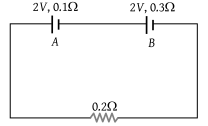(1) B will be zero

(2) A will be zero

(3) A and B will be 2V

(4) A will be > 2V and B will be < 2V

Concept Questions :-

Kirchoff's voltage law
High Yielding Test Series + Question Bank - NEET 2020

Difficulty Level:

The figure shows a network of currents. The magnitude of currents is shown here. The current i will be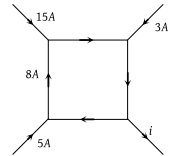(1) 3 A

(2) 13 A

(3) 23 A

(4) – 3 A

Concept Questions :-

Kirchoff's current law
High Yielding Test Series + Question Bank - NEET 2020

Difficulty Level:

A battery of e.m.f. E and internal resistance r is connected to a variable resistor R as shown here. Which one of the following is true :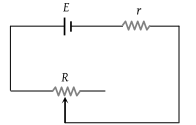(1) Potential difference across the terminals of the battery is maximum when R = r

(2) Power delivered to the resistor is maximum when R = r

(3) Current in the circuit is maximum when R = r

(4) Current in the circuit is maximum when R >> r

Concept Questions :-

Emf and terminal voltage
High Yielding Test Series + Question Bank - NEET 2020

Difficulty Level:

Consider the circuit given here with the following parameters E.M.F. of the cell = 12 V. Internal resistance of the cell = 2 Ω. Resistance R = 4 Ω Which one of the following statements in true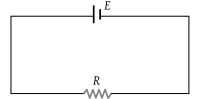(1) Rate of energy loss in the source is = 8 W

(2) Rate of energy conversion in the source is 16 W

(3) Power output in is = 8 W

(4) Potential drop across R is = 16 V

Concept Questions :-

Emf and terminal voltage
High Yielding Test Series + Question Bank - NEET 2020

Difficulty Level:

The current in the arm CD of the circuit will be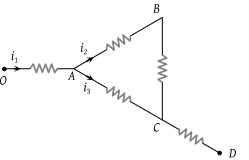(1) ${i}_{1}+{i}_{2}$

(2) ${i}_{2}+{i}_{3}$

(3) ${i}_{1}+{i}_{3}$

(4) ${i}_{1}-{i}_{2}+{i}_{3}$

Concept Questions :-

Kirchoff's current law
High Yielding Test Series + Question Bank - NEET 2020

Difficulty Level:

Two non-ideal identical batteries are connected in parallel. Consider the following statements :

(i) The equivalent e.m.f. is smaller than either of the two e.m.f.s

(ii) The equivalent internal resistance is smaller than either of the two internal resistances

(1) Both (i) and (ii) are correct

(2) (i) is correct but (ii) is wrong

(3) (ii) is correct but (i) is wrong

(4) Both (i) and (ii) are wrong

Concept Questions :-

Grouping of cells
High Yielding Test Series + Question Bank - NEET 2020

Difficulty Level:

Consider the circuit shown in the figure. The current I3 is equal to :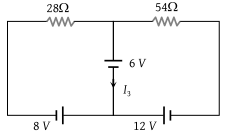(1) 5 amp

(2) 3 amp

(3) –3 amp

(4) –5/6 amp

Concept Questions :-

Kirchoff's voltage law
High Yielding Test Series + Question Bank - NEET 2020

Difficulty Level:

If ${V}_{AB}=4V$ in the given figure, then resistance X will be :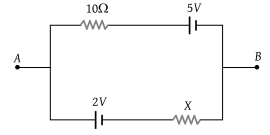(1) 5 Ω

(2) 10 Ω

(3) 15 Ω

(4) 20 Ω

Concept Questions :-

Kirchoff's voltage law